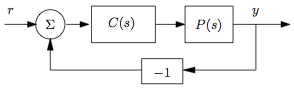# Frequency Domain Analysis

 Prev: Transfer Functions Chapter 9 - Loop Analysis Next: PID Control

In this chapter we study how how stability and robustness of closed loop systems can be determined by investigating how signals propagate around the feedback loop. The Nyquist stability theorem is a key result that provides a way to analyze stability and introduce measures of degrees of stability.

## Textbook Contents

• 1. Introduction
• 2. The Nyquist Criterion
• 3. Stability Margins
• 4. Bode's Relations
• 5. The Notion of Gain
• 7. Exercises

## Chapter Summary

This chapter describes the use of the Nyquist criterion for determining the stability of a system:

1. The loop transfer function of a feedback system represents the transfer function obtained by breaking the feedback loop and computing the resulting transfer function of the open loop system. For a simple feedback systemthe loop transfer function is given by2. The Nyquist criterion provides a way to check the stability of a closed loop system by looking at the properties of the loop transfer function. For a stable open loop system, the Nyquist criterion states that the system is stable if the contour of the loop transfer function plotted fromtohas no net encirclements of the pointwhen it is plotted on the complex plane.

3. The general Nyquist criterion uses the image of the loop transfer function applied to the Nyquist countour

File:Loopanal nyqcontour

The number of unstable poles of the closed loop system is given by the number of open loop unstable poles plus the number of clockwise encirclements of the point.

4. <p>Stability margins describe

5. Bode's relations

6. The gain of a system